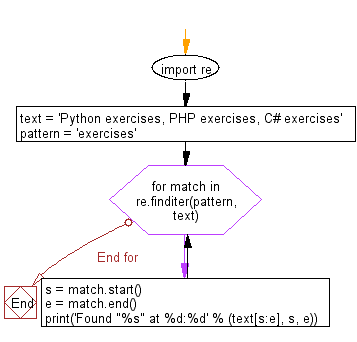﻿ Python Exercises: Find the occurrence and position of the substrings within a string - w3resource# Python: Find the occurrence and position of the substrings within a string

## Python Regular Expression: Exercise-22 with Solution

Write a Python program to find the occurrence and position of the substrings within a string.

Sample Solution:-

Python Code:

``````import re
text = 'Python exercises, PHP exercises, C# exercises'
pattern = 'exercises'
for match in re.finditer(pattern, text):
s = match.start()
e = match.end()
print('Found "%s" at %d:%d' % (text[s:e], s, e))
```
```

Sample Output:

```Found "exercises" at 7:16
Found "exercises" at 22:31
Found "exercises" at 36:45
```

Flowchart:## Visualize Python code execution:

The following tool visualize what the computer is doing step-by-step as it executes the said program:

Python Code Editor:

Have another way to solve this solution? Contribute your code (and comments) through Disqus.

What is the difficulty level of this exercise?

﻿

New Content: Composer: Dependency manager for PHP, R Programming